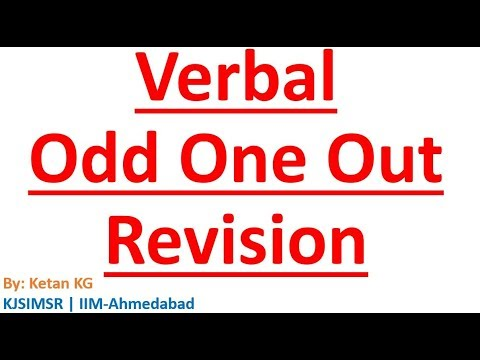Courses

Test: Odd Man Out- 1

20 Questions MCQ Test Business Mathematics and Logical Reasoning & Statistics | Test: Odd Man Out- 1

Description
Attempt Test: Odd Man Out- 1 | 20 questions in 20 minutes | Mock test for CA Foundation preparation | Free important questions MCQ to study Business Mathematics and Logical Reasoning & Statistics for CA Foundation Exam | Download free PDF with solutions
QUESTION: 1

Find the one which does not belong to that group ?

Solution:

3, 5, 9 and 7 are odd numbers, but not 4.

QUESTION: 2

Find the one which does not belong to that group ?

Solution:

37, 47, 67 and 17 are prime numbers but not 27.

QUESTION: 3

Find the one which does not belong to that group ?

Solution:

16, 36, 64 and 4 are perfect squares but not 28.

QUESTION: 4

Find the one which does not belong to that group ?

Solution:

41, 43, 47 and 53 are prime numbers, but not 57.

QUESTION: 5

Find the one which does not belong to the group ?

Solution:

36 = 62, 49 = 72, 64 = 82, 81 = 92 and 100 = 102.

36, 64, 81 and 100 are squares of composite numbers, but 49 is a square of prime number 7.

QUESTION: 6

Find the one which does not belong to that group ?

Solution:

8 = 23, 27 = 33, 64 = 43, 125 = 53 and 343 = 73.

8, 27, 125 and 343 are cubes of prime numbers but not 64.

QUESTION: 7

Find the one which does not belong to that group ?

Solution:

343 = 73, 121 = 112, 1331 = 113, 2197 = 133 and 125 = 53.

343, 1331, 2197 and 125 are perfect cubes, but not 121.

QUESTION: 8

Find the one which does not belong to that group ?

Solution:

48, 75, 84 and 57 are divisible by 3 but not 35.

QUESTION: 9

Find the one which does not belong to that group ?

Solution:

42624, 37573, 74347 and 93339 are palindromes but not 84284.

QUESTION: 10

Find the one which does not belong to that group ?

Solution:

27, 36, 72 and 45 are divisible by 9, but not 30.

QUESTION: 11

Find the one which does not belong to that group ?

Solution:

Except 4242, all other numbers are divisible by 11.

QUESTION: 12

Find the one which does not belong to that group ?

Solution:

20 = 42 + 4, 42 = 62 + 6, 58 = 72 + 9, 72 = 82 + 8 and 90 = 92 + 9.

20, 42, 72 and 90 can be expressed in n2 + n form but not 58.

QUESTION: 13

Find the one which does not belong to that group ?

Solution:

30 = 33 + 3, 630 = 54 + 5, 10 = 23 + 2, 520 = 83 + 8 and 130 = 53 + 5.

30, 10, 130 and 520 can be expressed as n3 + n but not 630.

QUESTION: 14

Find the one which does not belong to that group ?

Solution:

The sum of the digits in 508, 328, 706 and 148 is 13, but not in 608.

QUESTION: 15

Find the one which does not belong to that group ?

Solution:

Except in 862, in all other numbers sum of first two digits is same as the last digit.

QUESTION: 16

Find the one which does not belong to that group ?

Solution:

525 => 5 52,

39 => 3 32,

24 => 2 22,

636 => 6 62. The above pattern is not followed in 426.

QUESTION: 17

Find the one which does not belong to that group ?

Solution:

11 = 1 13, 28 = 2 23, 327 = 3 33, 416 = 4 42 and 5125 = 5 53

Except 416, other numbers follow similar pattern.

QUESTION: 18

Find the one which does not belong to that group ?

Solution:

Except 132, other numbers are odd numbers.

QUESTION: 19

Find the one which does not belong to that group ?

Solution:

Except 110, other numbers are divisible by 4.

QUESTION: 20

Find the one which does not belong to that group ?

Solution:

A, E, I and O are vowels, while R is a consonant.Use Code STAYHOME200 and get INR 200 additional OFF Use Coupon Code

Track your progress, build streaks, highlight & save important lessons and more!

Similar ContentRelated tests